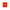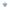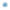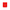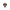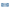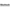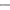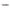# Plenary, Invited, Prize and Special Lectures

## ICM Plenary Lectures

An invitation to give a plenary lecture to the thousands of ICM participants is considered a special distinction in the mathematics profession. The Program Committee selects the plenary lectures for the ICM very carefully. Traditionally, they occupy the morning half of the program, drawing the attention of all ICM participants and thousands of other mathematicians via live stream. Each lecture begins with the introduction of the speaker by another world-renowned scholar who chairs the session.

The list of plenary speakers of the ICM 2022 is published on this page.

The ICM website will have special supplementary materials for each plenary lecture. These materials will help attendees learn more about new developments and areas of mathematics that may be off the beaten track. Lectures will be published in the ICM proceedings and freely available online.

## Prize and Special Lectures

All IMU prize winners address the whole ICM community in their prize lecture, giving the community the opportunity to meet the winners and learn more about their exciting research. The prize lectures follow the same format as the plenary lectures. Special lectures such as the Abel Lecture, given by a recent recipient of the Abel Prize, follow a similar format.

## ICM Invited Lectures

Traditionally, the invited lectures occupy the afternoon portion of the program and are organized into sections. For the St. Petersburg ICM, the following twenty sections have been listed by the ICM Structure Committee.

Name
№ of lectures
Description
Connection with sections
1
Logic
3–5
Model theory. Proof theory. Recursion theory (Computability theory). Set theory. Applications.
2, 3, 4, 8, 13, 14
2
Algebra
3–6
Groups (finite, infinite, algebraic) and their representations. Rings (both commutative and non-commutative), fields and modules. General algebraic structures, algebraic K-theory, category theory. Computational aspects of algebra and applications.
2, 3, 4, 8, 13, 14
3
Number Theory
8–11
Algebraic number theory. Galois groups of local and global fields and their representations. Arithmetic of algebraic varieties and Diophantine equations. Geometry of numbers, Diophantine approximation, and transcendental numbers. P-adic analysis. Modular and automorphic forms, modular curves, and Shimura varieties. Langlands program. Zeta and L-functions. Analytic, additive and probabilistic number theory. Computational number theory and applications. Relations with logic and with physics.
1, 2, 4, 7, 9, 11, 12, 13, 14
4
Algebraic and Complex Geometry
8–11
Algebraic varieties, their cycles, cohomologies, and motives. Schemes and stacks. Geometric aspects of commutative algebra. Arithmetic geometry. Rational points. Low-dimensional and special varieties. Singularities. Birational geometry and minimal models. Moduli spaces and enumerative geometry. Transcendental methods and topology of algebraic varieties. Complex differential geometry, Kähler manifolds and Hodge theory. Relations with mathematical physics and representation theory. Computational methods. Real algebraic and analytic sets. p-adic geometry. D-modules and (iso)crystals. Tropical geometry. Derived categories and non-commutative geometry.
1, 2, 3, 5, 6, 7, 8, 11, 13, 14
5
Geometry
8–11
Local and global differential geometry. Geometric partial differential equations and geometric flows. Geometric structures on manifolds. Riemannian and metric geometry. Kähler geometry. Geometric aspects of group theory. Symplectic and contact manifolds. Convex geometry. Discrete geometry.
2, 4, 6, 7, 8, 9, 10, 11, 12, 16, 17
6
Topology
7–10
Algebraic, differential and geometric topology. Surgery and diffeomorphism groups of manifolds. Homotopy theory, including motivic homotopy and K-theory. Operads and higher categories. Floer and gauge theories. Lowdimensional manifolds including knot theory. Moduli spaces. Symplectic and contact manifolds. Aspects of quantum field theory.
2, 3, 4, 5, 7, 8, 9, 11
7
Lie Theory and Generalizations
6–9
Structure, geometry, and representations of Lie groups, algebraic groups, and their various generalizations. Related geometric and algebraic objects, e.g., symmetric spaces, buildings, and other Lie theoretic varieties, vertex operator algebras, quantum groups. Lattices and other discrete subgroups of Lie groups, and their actions on geometric objects. Non-commutative harmonic analysis. Geometric methods in representation theory
2, 3, 4, 5, 6, 8, 9, 11, 12, 13
8
Analysis
9–12
Classical analysis. Real and Complex analysis in one and several variables, potential theory, quasiconformal mappings. Harmonic, Fourier, and time-frequency analysis. Linear and non-linear functional analysis, operator algebras, Banach algebras, Banach spaces. Non-commutative geometry, free probability, analysis of random matrices. High-dimensional and asymptotic geometric analysis. Metric geometry and applications. Geometric measure theory
5, 6, 7, 9, 10, 11, 12, 13, 14, 15, 16, 17
9
Dynamics
8–11
Topological and symbolic dynamics. Smooth dynamical systems, including those derived from ordinary differential equations. Hamiltonian systems and dynamical systems of geometric origin. One-dimensional, holomorphic and arithmetic dynamics. Dynamics on moduli spaces. Ergodic theory, including applications to combinatorics and combinatorial number theory. Actions of discrete groups and rigidity theory. Homogenous dynamics, including applications to number theory. Infinite dimensional dynamical systems and partial differential equations.
5, 8, 9, 11, 12, 15, 16, 17, 18
10
Partial Differential Equations
8–11
Solvability, regularity, stability and other qualitative and quantitative properties of linear and non-linear equations and systems. Asymptotics. Spectral theory, scattering, inverse problems, deterministic and stochastic control theory, stochastic differential equations. Nonlocal equations, free boundary problems, calculus of variations, kinetic equations. Optimal transportation. Homogenization and multi-scale problems. Approximate solutions and perturbation problems. Relations to many applications.
5, 8, 9, 11, 12, 15, 16, 17, 18
11
Mathematical Physics
8–11
Dynamical systems, including integrable systems. Equilibrium and non-equilibrium statistical mechanics, including interacting particle systems. Partial differential equations including fluid dynamics, wave equation, Boltzmann equation and material science. General relativity. Stochastic models and probabilistic methods including random matrices and stochastic (partial) differential equations. Algebraic methods, including operator algebras, representation theory and algebraic aspects of Quantum Field Theory. Quantum mechanics and spectral theory, including quantum chaos. Quantum information and computation. Quantum many-body theory and condensed matter physics. Quantum field theory including gauge theories and conformal field theory. Geometry and topology in physics including string theory and quantum gravity.
2, 4, 5, 6, 7, 8, 9, 10, 12
12
Probability
7–10
Stochastic analysis, Stochastic PDEs, Markov processes. Interacting particle systems, Random media. Random matrices and random graphs. Conformally invariant models, random growth models, exactly solvable models. Branching processes. Rough paths, regularity structures. Stochastic networks, Stochastic geometry. Applications in Statistics, Data Science, Computer Science, Physics, and Life Sciences.
2, 3, 5, 7, 8, 9, 10, 11, 13, 14, 15, 16, 17, 18
13
Combinatorics
7–10
Combinatorial structures. Enumeration: exact and asymptotic. Graph theory. Probabilistic and extremal combinatorics. Designs and finite geometries. Algebraic combinatorics. Topological and analytical techniques in combinatorics. Combinatorial geometry. Combinatorial number theory. Additive combinatorics. Polyhedral combinatorics and combinatorial optimization.
1, 2, 3, 4, 6, 7, 8, 9, 12, 14
14
Mathematics of Computer Science
5–7
Computational complexity theory, Design and analysis of algorithms. Automata and Formal languages. Cryptography. Randomness and pseudorandomness. Computational learning. Optimization. Algorithmic game theory. Distributed systems and networks. Coding and Information theory. Semantics and verification of programs. Symbolic and numeric computation. Quantum computing and information. Algorithmic and computational aspects in mathematics. Computational models and problems in the natural and social sciences.
1, 2, 3, 4, 5, 6, 7, 8, 9, 10, 11, 12, 13, 14, 15, 16, 17, 18
15
Numerical Analysis and Scientific Computing
5–7
Design of numerical algorithms and analysis of their accuracy, stability, convergence and complexity for a wide class of (complex) problems with interests in applications. Numerical methods for high dimensional problems. Multiscale problems and probabilistic numerical methods. Approximation theory andcomputational aspects of harmonic analysis. Numerical reduction and uncertainty quantification. Numerical solution of algebraic, functional, stochastic, differential, and integro-differential equations.
8, 9, 10, 12, 14, 16, 17, 18
16
Control Theory and Optimization
5–7
Minimization problems. Controllability, observability, stability. Robotics. Stochastic systems and control. Optimal control. Optimal design, shape design. Linear, non-linear, integer, and stochastic programming. Inverse problems. Applications.
9, 10, 12, 13, 14, 15, 17, 18
17
Statistics and Data Analysis
8–11
All areas of statistics, including inference, parametric and nonparametric statistics, together with all branches of mathematics for data science, where data science includes machine learning, signal and image processing, data generation, data representation, and their applications.
2, 5, 8, 11, 12, 14, 15, 16, 18
18
Stochastic and Differential Modelling
4–6
The mathematical development of stochastic and deterministic differential modelling, and applications to such fields as biology, chemistry, medicine, material science, finance, and social network modelling. Deterministic and stochastic systems of any (possibly high) dimension, and at several scales (multiscale modelling). Tools for model reduction, calibration, uncertainty quantification and data assimilation.
9, 10, 11, 12, 15, 17
19
Mathematical Education and Popularization of Mathematics
2+3 panels
Range of research and key issues in mathematics education, from elementary school to higher education. Modern developments in effective popularization of mathematics, from publications, to museums, to online communication.
17, 20
20
History of Mathematics
3
Historical studies of all of the mathematical sciences in all periods and all cultural settings.